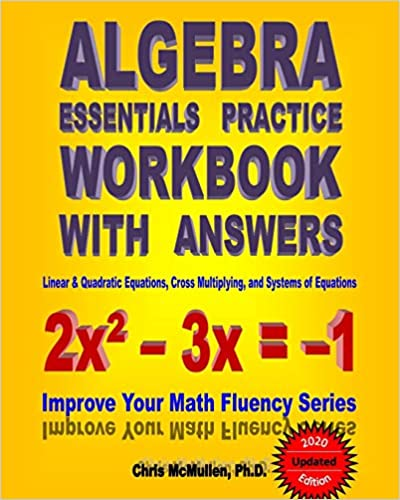## Change Language# Algebra Essentials Practice Workbook with Answers: Linear & Quadratic Equations, Cross Multiplying, and Systems of Equations: Improve Your Math FluencyAuthor(s): Chris McMullen Collection: Improve Your Math Fluency Series Publisher: CreateSpace Independent Publishing Platform Year: July 12th 2010 Language: English Pages: 207 pages Size: 1.96 MB Extension: PDF

[tab] [content title="Description"]
CONTENTS: This Algebra Essentials Practice Workbook with Answers provides ample practice for developing fluency in very fundamental algebra skills - in particular, how to solve standard equations for one or more unknowns. These algebra 1 practice exercises are relevant for students of all levels - from grade 7 thru college algebra. This workbook is conveniently divided up into seven chapters so that students can focus on one algebraic method at a time. Skills include solving linear equations with a single unknown (with a separate chapter dedicated toward fractional coefficients), factoring quadratic equations, using the quadratic formula, cross multiplying, and solving systems of linear equations. Not intended to serve as a comprehensive review of algebra, this workbook is instead geared toward the most essential algebra skills. An introduction describes how parents and teachers can help students make the most of this workbook. Students are encouraged to time and score each page. In this way, they can try to have fun improving on their records, which can help lend them confidence in their math skills.

PRACTICE: With no pictures, this workbook is geared strictly toward learning the material and developing fluency through practice.

EXAMPLES: Each section begins with a few pages of instructions for how to solve the equations followed by a few examples. These examples should serve as a useful guide until students are able to solve the problems independently.

ANSWERS: Answers to 100% of the exercises are tabulated at the back of the book. This helps students develop confidence and ensures that students practice correct techniques, rather than practice making mistakes. All answers from the updated edition have been verified both by computer and independently by an international math whiz.

PHOTOCOPIES: The copyright notice permits parents/teachers who purchase one copy or borrow one copy from a library to make photocopies for their own children/students only. This is very convenient if you have multiple children/students or if a child/student needs additional practice.

NOTE: The answer key uses standard form. Fractions are expressed as reduced fractions, denominators are rationalized, and perfect squares are factored out of square roots. For example, if you receive an answer of 1 over root 8, the answer key will instead show root 2 over 4; both answers are the same, but the answer key uses standard form.
[/content] [content title="Content"] [/content] [content title="About the author"]Chris McMullen is a physics instructor at Northwestern State University of Louisiana. He earned his Ph.D. in phenomenological high-energy physics (particle physics) from Oklahoma State University in 2002. Originally from California, he earned his Master's degree from California State University, Northridge, where his thesis was in the field of electron spin resonance. As a physics teacher, Dr. McMullen observed that many students lack fluency in fundamental math skills. In an effort to help students of all ages and levels master basic math skills, he has published this Improve Your Math Fluency Series of math workbooks on arithmetic, fractions, and algebra. [/content] [/tab]